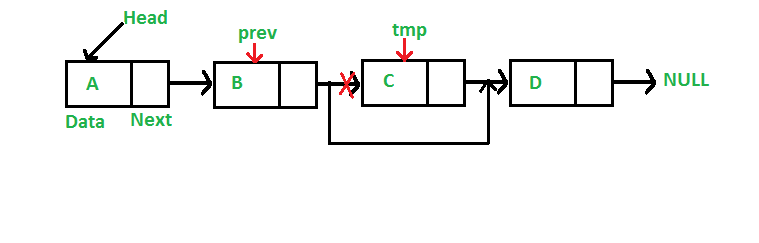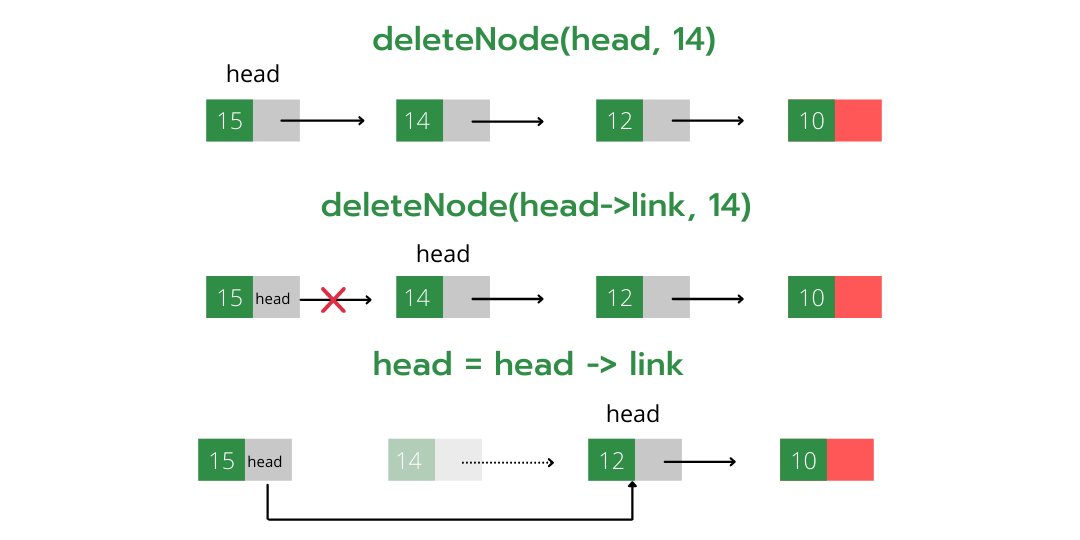# Linked List | Set 3 (Deleting a node)

• Difficulty Level : Easy
• Last Updated : 22 Nov, 2021

We have discussed Linked List Introduction and Linked List Insertion in previous posts on a singly linked list.
Let us formulate the problem statement to understand the deletion process. Given a ‘key’, delete the first occurrence of this key in the linked list

Iterative Method:
To delete a node from the linked list, we need to do the following steps.
1) Find the previous node of the node to be deleted.
2) Change the next of the previous node.
3) Free memory for the node to be deleted.

Attention reader! Don’t stop learning now. Get hold of all the important DSA concepts with the DSA Self Paced Course at a student-friendly price and become industry ready.  To complete your preparation from learning a language to DS Algo and many more,  please refer Complete Interview Preparation Course.

In case you wish to attend live classes with experts, please refer DSA Live Classes for Working Professionals and Competitive Programming Live for Students.Since every node of the linked list is dynamically allocated using malloc() in C, we need to call free() for freeing memory allocated for the node to be deleted.

## C++

 `// A complete working C++ program to``// demonstrate deletion in singly``// linked list with class``#include ``using` `namespace` `std;` `// A linked list node``class` `Node{``public``:``    ``int` `data;``    ``Node* next;``};` `// Given a reference (pointer to pointer)``// to the head of a list and an int,``// inserts a new node on the front of the``// list.``void` `push(Node** head_ref, ``int` `new_data)``{``    ``Node* new_node = ``new` `Node();``    ``new_node->data = new_data;``    ``new_node->next = (*head_ref);``    ``(*head_ref) = new_node;``}` `// Given a reference (pointer to pointer)``// to the head of a list and a key, deletes``// the first occurrence of key in linked list``void` `deleteNode(Node** head_ref, ``int` `key)``{``    ` `    ``// Store head node``    ``Node* temp = *head_ref;``    ``Node* prev = NULL;``    ` `    ``// If head node itself holds``    ``// the key to be deleted``    ``if` `(temp != NULL && temp->data == key)``    ``{``        ``*head_ref = temp->next; ``// Changed head``        ``delete` `temp;            ``// free old head``        ``return``;``    ``}` `    ``// Else Search for the key to be deleted,``    ``// keep track of the previous node as we``    ``// need to change 'prev->next' */``      ``else``    ``{``    ``while` `(temp != NULL && temp->data != key)``    ``{``        ``prev = temp;``        ``temp = temp->next;``    ``}` `    ``// If key was not present in linked list``    ``if` `(temp == NULL)``        ``return``;` `    ``// Unlink the node from linked list``    ``prev->next = temp->next;` `    ``// Free memory``    ``delete` `temp;``    ``}``}` `// This function prints contents of``// linked list starting from the``// given node``void` `printList(Node* node)``{``    ``while` `(node != NULL)``    ``{``        ``cout << node->data << ``" "``;``        ``node = node->next;``    ``}``}` `// Driver code``int` `main()``{``    ` `    ``// Start with the empty list``    ``Node* head = NULL;` `    ``// Add elements in linked list``    ``push(&head, 7);``    ``push(&head, 1);``    ``push(&head, 3);``    ``push(&head, 2);` `    ``puts``(``"Created Linked List: "``);``    ``printList(head);` `    ``deleteNode(&head, 1);``    ``puts``(``"\nLinked List after Deletion of 1: "``);``    ` `    ``printList(head);``    ` `    ``return` `0;``}` `// This code is contributed by ac121102`

## C

 `// A complete working C program``// to demonstrate deletion in``// singly linked list``#include ``#include ` `// A linked list node``struct` `Node {``    ``int` `data;``    ``struct` `Node* next;``};` `/* Given a reference (pointer to pointer) to the head of a``   ``list and an int, inserts a new node on the front of the``   ``list. */``void` `push(``struct` `Node** head_ref, ``int` `new_data)``{``    ``struct` `Node* new_node``        ``= (``struct` `Node*)``malloc``(``sizeof``(``struct` `Node));``    ``new_node->data = new_data;``    ``new_node->next = (*head_ref);``    ``(*head_ref) = new_node;``}` `/* Given a reference (pointer to pointer) to the head of a``   ``list and a key, deletes the first occurrence of key in``   ``linked list */``void` `deleteNode(``struct` `Node** head_ref, ``int` `key)``{``    ``// Store head node``    ``struct` `Node *temp = *head_ref, *prev;` `    ``// If head node itself holds the key to be deleted``    ``if` `(temp != NULL && temp->data == key) {``        ``*head_ref = temp->next; ``// Changed head``        ``free``(temp); ``// free old head``        ``return``;``    ``}` `    ``// Search for the key to be deleted, keep track of the``    ``// previous node as we need to change 'prev->next'``    ``while` `(temp != NULL && temp->data != key) {``        ``prev = temp;``        ``temp = temp->next;``    ``}` `    ``// If key was not present in linked list``    ``if` `(temp == NULL)``        ``return``;` `    ``// Unlink the node from linked list``    ``prev->next = temp->next;` `    ``free``(temp); ``// Free memory``}` `// This function prints contents of linked list starting``// from the given node``void` `printList(``struct` `Node* node)``{``    ``while` `(node != NULL) {``        ``printf``(``" %d "``, node->data);``        ``node = node->next;``    ``}``}` `// Driver code``int` `main()``{``    ``/* Start with the empty list */``    ``struct` `Node* head = NULL;` `    ``push(&head, 7);``    ``push(&head, 1);``    ``push(&head, 3);``    ``push(&head, 2);` `    ``puts``(``"Created Linked List: "``);``    ``printList(head);``    ``deleteNode(&head, 1);``    ``puts``(``"\nLinked List after Deletion of 1: "``);``    ``printList(head);``    ``return` `0;``}`

## Java

 `// A complete working Java program``// to demonstrate deletion``// in singly linked list``class` `LinkedList {``    ``Node head; ``// head of list` `    ``/* Linked list Node*/``    ``class` `Node {``        ``int` `data;``        ``Node next;``        ``Node(``int` `d)``        ``{``            ``data = d;``            ``next = ``null``;``        ``}``    ``}` `    ``/* Given a key, deletes the first``       ``occurrence of key in``     ``* linked list */``    ``void` `deleteNode(``int` `key)``    ``{``        ``// Store head node``        ``Node temp = head, prev = ``null``;` `        ``// If head node itself holds the key to be deleted``        ``if` `(temp != ``null` `&& temp.data == key) {``            ``head = temp.next; ``// Changed head``            ``return``;``        ``}` `        ``// Search for the key to be deleted, keep track of``        ``// the previous node as we need to change temp.next``        ``while` `(temp != ``null` `&& temp.data != key) {``            ``prev = temp;``            ``temp = temp.next;``        ``}` `        ``// If key was not present in linked list``        ``if` `(temp == ``null``)``            ``return``;` `        ``// Unlink the node from linked list``        ``prev.next = temp.next;``    ``}` `    ``/* Inserts a new Node at front of the list. */``    ``public` `void` `push(``int` `new_data)``    ``{``        ``Node new_node = ``new` `Node(new_data);``        ``new_node.next = head;``        ``head = new_node;``    ``}` `    ``/* This function prints contents of linked list starting``       ``from the given node */``    ``public` `void` `printList()``    ``{``        ``Node tnode = head;``        ``while` `(tnode != ``null``) {``            ``System.out.print(tnode.data + ``" "``);``            ``tnode = tnode.next;``        ``}``    ``}` `    ``/* Driver program to test above functions. Ideally this``    ``function should be in a separate user class. It is kept``    ``here to keep code compact */``    ``public` `static` `void` `main(String[] args)``    ``{``        ``LinkedList llist = ``new` `LinkedList();` `        ``llist.push(``7``);``        ``llist.push(``1``);``        ``llist.push(``3``);``        ``llist.push(``2``);` `        ``System.out.println(``"\nCreated Linked list is:"``);``        ``llist.printList();` `        ``llist.deleteNode(``1``); ``// Delete node with data 1` `        ``System.out.println(``            ``"\nLinked List after Deletion of 1:"``);``        ``llist.printList();``    ``}``}`

## Python3

 `# A complete working Python3 program to``# demonstrate deletion in singly``# linked list with class` `# Node class``class` `Node:` `    ``# Constructor to initialize the node object``    ``def` `__init__(``self``, data):``        ``self``.data ``=` `data``        ``self``.``next` `=` `None` `class` `LinkedList:` `    ``# Function to initialize head``    ``def` `__init__(``self``):``        ``self``.head ``=` `None` `    ``# Function to insert a new node at the beginning``    ``def` `push(``self``, new_data):``        ``new_node ``=` `Node(new_data)``        ``new_node.``next` `=` `self``.head``        ``self``.head ``=` `new_node` `    ``# Given a reference to the head of a list and a key,``    ``# delete the first occurrence of key in linked list``    ``def` `deleteNode(``self``, key):``        ` `        ``# Store head node``        ``temp ``=` `self``.head` `        ``# If head node itself holds the key to be deleted``        ``if` `(temp ``is` `not` `None``):``            ``if` `(temp.data ``=``=` `key):``                ``self``.head ``=` `temp.``next``                ``temp ``=` `None``                ``return` `        ``# Search for the key to be deleted, keep track of the``        ``# previous node as we need to change 'prev.next'``        ``while``(temp ``is` `not` `None``):``            ``if` `temp.data ``=``=` `key:``                ``break``            ``prev ``=` `temp``            ``temp ``=` `temp.``next` `        ``# if key was not present in linked list``        ``if``(temp ``=``=` `None``):``            ``return` `        ``# Unlink the node from linked list``        ``prev.``next` `=` `temp.``next` `        ``temp ``=` `None`  `    ``# Utility function to print the linked LinkedList``    ``def` `printList(``self``):``        ``temp ``=` `self``.head``        ``while``(temp):``            ``print` `(``" %d"` `%``(temp.data)),``            ``temp ``=` `temp.``next`  `# Driver program``llist ``=` `LinkedList()``llist.push(``7``)``llist.push(``1``)``llist.push(``3``)``llist.push(``2``)` `print` `(``"Created Linked List: "``)``llist.printList()``llist.deleteNode(``1``)``print` `(``"\nLinked List after Deletion of 1:"``)``llist.printList()` `# This code is contributed by Nikhil Kumar Singh (nickzuck_007)`

## C#

 `// A complete working C# program``// to demonstrate deletion in``// singly linked list``using` `System;``class` `GFG{` `// Head of list``Node head;` `// Linked list Node``public` `class` `Node``{``  ``public` `int` `data;``  ``public` `Node next;``  ``public` `Node(``int` `d)``  ``{``    ``data = d;``    ``next = ``null``;``  ``}``}` `// Given a key, deletes the first``// occurrence of key in linked list``void` `deleteNode(``int` `key)``{``  ``// Store head node``  ``Node temp = head, prev = ``null``;` `  ``// If head node itself holds``  ``// the key to be deleted``  ``if` `(temp != ``null` `&&``      ``temp.data == key)``  ``{``    ``// Changed head``    ``head = temp.next;``    ``return``;``  ``}` `  ``// Search for the key to be``  ``// deleted, keep track of the``  ``// previous node as we need``  ``// to change temp.next``  ``while` `(temp != ``null` `&&``         ``temp.data != key)``  ``{``    ``prev = temp;``    ``temp = temp.next;``  ``}   ` `  ``// If key was not present``  ``// in linked list``  ``if` `(temp == ``null``)``    ``return``;` `  ``// Unlink the node from linked list``  ``prev.next = temp.next;``}` `// Inserts a new Node at``// front of the list.``public` `void` `Push(``int` `new_data)``{``  ``Node new_node = ``new` `Node(new_data);``  ``new_node.next = head;``  ``head = new_node;``}` `// This function prints contents``// of linked list starting from``// the given node``public` `void` `printList()``{``  ``Node tnode = head;``  ``while` `(tnode != ``null``)``  ``{``    ``Console.Write(tnode.data + ``" "``);``    ``tnode = tnode.next;``  ``}``}``  ` `// Driver code``public` `static` `void` `Main(String[] args)``{``  ``GFG llist = ``new` `GFG();` `  ``llist.Push(7);``  ``llist.Push(1);``  ``llist.Push(3);``  ``llist.Push(2);` `  ``Console.WriteLine(``"\nCreated Linked list is:"``);``  ``llist.printList();` `  ``// Delete node with data 1``  ``llist.deleteNode(1);` `  ``Console.WriteLine(``"\nLinked List after Deletion of 1:"``);``  ``llist.printList();``}``}` `// This code is contributed by Rajput-Ji`

## Javascript

 ``
Output
```Created Linked List:
2  3  1  7
Linked List after Deletion of 1:
2  3  7```

Recursive Method:

To delete a node of a linked list recursively we need to do the following steps.

1. We pass node* (node pointer) as a reference to the function (as in node* &head)

2. Now since the current node pointer is derived from the previous node’s next (which is passed by reference) so now if the value of the current node pointer is changed, the previous next node’s value also gets changed which is the required operation while deleting a node (i.e points previous node’s next to current node’s (containing key) next).

3. Find the node containing the given value.

4. Store this node to deallocate it later using the free() function.

5. Change this node pointer so that it points to its next and by performing this previous node’s next also gets properly linked.Below is the implementation of the above approach.

## C++

 `// C++ program to delete a node in``// singly linked list recursively` `#include ``using` `namespace` `std;` `struct` `node {``    ``int` `info;``    ``node* link = NULL;``    ``node() {}``    ``node(``int` `a)``        ``: info(a)``    ``{``    ``}``};` `/*``Deletes the node containing 'info' part as val and``alter the head of the linked list (recursive method)``*/``void` `deleteNode(node*& head, ``int` `val)``{``    ` `    ``// Check if list is empty or we``    ``// reach at the end of the``    ``// list.``    ``if` `(head == NULL) {``        ``cout << ``"Element not present in the list\n"``;``        ``return``;``    ``}``    ``// If current node is the node to be deleted``    ``if` `(head->info == val) {``        ``node* t = head;``        ``head = head->link; ``// If it's start of the node head``                           ``// node points to second node``        ``delete` `(t); ``// Else changes previous node's link to``                    ``// current node's link``        ``return``;``    ``}``    ``deleteNode(head->link, val);``}` `// Utility function to add a``// node in the linked list``// Here we are passing head by``// reference thus no need to``// return it to the main function``void` `push(node*& head, ``int` `data)``{``    ``node* newNode = ``new` `node(data);``    ``newNode->link = head;``    ``head = newNode;``}` `// Utility function to print``// the linked list (recursive``// method)``void` `print(node* head)``{``    ` `    ``// cout<info << ``' '``;``    ``print(head->link);``}` `int` `main()``{``    ``// Starting with an empty linked list``    ``node* head = NULL;` `    ``// Adds new element at the``    ``// beginning of the list``    ``push(head, 10);``    ``push(head, 12);``    ``push(head, 14);``    ``push(head, 15);` `    ``// original list``    ``print(head);` `    ``deleteNode(head, 20); ``// Call to delete function``    ``print(head); ``// 20 is not present thus no change in the``                 ``// list` `    ``deleteNode(head, 10);``    ``print(head);` `    ``deleteNode(head, 14);``    ``print(head);` `    ``return` `0;``}`
```Element not present in the list
15 14 12 10
15 14 12
15 12 ```